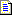English (English) - Change language

# Hypotheses for Character String elements

If a regular expression has been specified in the properties of an element, the program will look for any strings in the search area that meet the conditions in the regular expression. If no regular expression has been specified, the program uses the user-defined alphabets.

The program considers all the text objects which horizontally intersect the search area (vertically the objects must fit within the search area in their entirety). The text objects are then grouped into lines. Lines are built left to right. The program stops building a line when the maximum length of space (set in the Max space length property) is exceeded.

In the resulting lines, the program identifies character strings, each of which contains characters only from one of the user-defined alphabets. In a similar fashion, the program divides lines into fragments.

Next, the program formulates a hypothesis for each of the fragments. Depending on whether the Allow embedded hypotheses option is selected or not, hypothesis are formulated on two different principles.

Suppose the program detected three fragments at a previous stage. If the Allow embedded hypotheses option is selected, hypotheses are formulated as follows:

hypothesis 1: fragment 1

hypothesis 2: fragment 1 + fragment 2

hypothesis 3: fragment 1 + fragment 2 + fragment 3

hypothesis 4: fragment 2

hypothesis 5: fragment 2 + fragment 3

hypothesis 6: fragment 3

For each hypothesis, the program will check that the portion of characters of each alphabet does not exceed the value set in the Percentage of alphabet characters field. Similarly, the program checks that the percentage of non-alphabet characters does not exceed the value set in the Percentage of non-alphabet characters field. If it least one of the checks fails, no hypothesis is formulated.

If the Allow embedded hypotheses is not selected, the embedded hypotheses in the list above will be discarded. Embedded hypotheses are those which are contained within another hypothesis in the list above. If the checks were successful for all of the hypotheses, only the following hypothesis will remain: fragment 1 + fragment 2 + fragment 3.

Thus, if the Allow embedded hypotheses option is not selected, the program formulates hypotheses of maximum length which meet all of the conditions. Even though embedded hypotheses are excluded, hypotheses may intersect. This may be a stand-alone character or word, or a string of characters which are part of other hypotheses but for which no separate hypotheses have been formulated. For example, the program may formulate two hypotheses (i.e. two strings) - one ending in a certain word or phrase and another starting with that word or phrase.

E.g.

hypothesis 1: fragment 1 + fragment 2

hypothesis 2: fragment 2 + fragment 3

Once all the possible hypotheses have been generated, the program calculates the Pre-search quality for each (this is an estimate of how well a hypothesis meets the search constraints set in the Properties dialog box on the Character String tab and on the Advanced tab in the Advanced pre-search relations field). At this stage, the quality is calculated based on whether the length of the hypothesis in characters falls within the fuzzy interval specified in the Character count property, on whether the length of the total gap in the line falls within the fuzzy interval specified in TotalGapLength, and on whether the number of words in the line fall within the fuzzy interval specified in the Word count property.

The overall quality of a hypothesis is calculated by multiplying all the qualities.

A Character String hypothesis has the following properties:

 Property Description Element name The full name of the element. Page The number of the page on which the element was detected. Surrounding rect The coordinates of the rectangle which surrounds the region of the hypothesis. Width The width of the region of the hypothesis. Height The height of the region of the hypothesis. Text The characters in the hypothesis. Detected Shows whether the object described by the element has been found (true) or whether a null hypothesis has been formulated (false). From the best path Shows whether the found hypothesis belongs to the best path in the tree of hypotheses (true) or not (false). Pre-search quality How well the hypothesis matches the properties of the element specified by the settings in the Properties dialog box and by the code in the Advanced pre-search relations field. Post-search quality The quality of the hypothesis after the conditions in the Advanced post-search relations field have been applied. Chain quality The quality of the chain of hypotheses, from the first subelement of the group to the current subelement. Chain quality is calculated by multiplying the qualities of all the subelements in the chain and is used to compare rival chains of hypotheses.

#### More:Character StringSearch areaAdditional search constraints

12/1/2020 7:03:59 AM